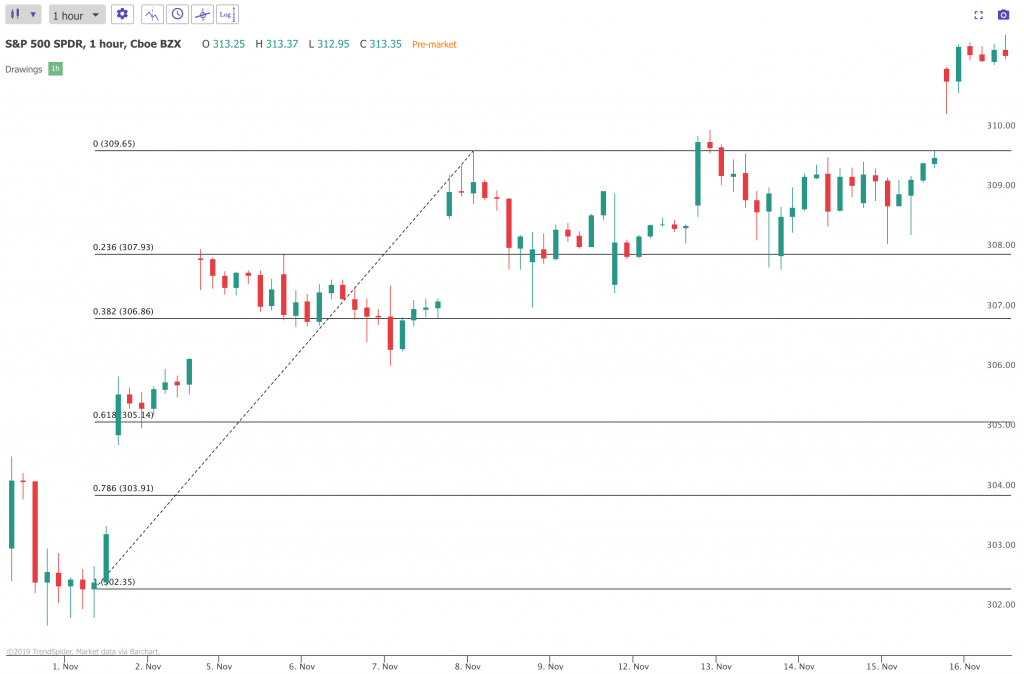# Automated Fibonacci Retracements

Leonardo Fibonacci discovered the Fibonacci sequence in 1202 AD, but couldn’t have appreciated how far and wide the discovery would be felt around the world.

Many traders use Fibonacci retracements and extensions to determine support and resistance levels, as well as stop-loss, take-profit and entry points. Despite its wide use, many traders misuse Fibonacci tools by incorrectly selecting the two points used to draw the sequence. This is especially challenging in a volatile market.

TrendSpider automatically calculates the most meaningful Fibonacci connection points on each timeframe and draws a retracement for you. In addition, you can plot Fibonacci sequences across different timeframes, providing broader insights that are difficult to glean elsewhere.You can create Fibonacci retracements in a few easy steps:

1. Select the Fibonacci tool from the drawing panel or press Alt + F.
2. Click on the starting point for the Fibonacci retracement.
3. Click on the ending point for the Fibonacci retracement.

You can customize the Fibonacci retracement by right-clicking on the annotation and selecting Properties.

Note: When using the automated Fibonacci tool, a manual Fibonacci is initially created and locked in place. To customize, you can change your manual Fibonacci properties and then turn Auto-Fibonacci on. It will then use the same colors and inputs that you customized. The manual Fibonacci drawing tool inherits the colored center automatically similar to what is seen with our Auto-Fibonacci tool.

Mar 5, 2021